Home | | Transforms and Partial Differential Equations | Solution of a Partial Differential Equation

# Solution of a Partial Differential Equation

A solution or integral of a partial differential equation is a relation connecting the dependent and the independent variables which satisfies the given differential equation.

SOLUTIONS OF A PARTIAL DIFFERENTIAL EQUATION

A solution or integral of a partial differential equation is a relation connecting the dependent and the independent variables which satisfies the given differential equation. A partial differential equation can result both from elimination of arbitrary constants and from elimination of arbitrary functions as explained in section 1.2. But, there is a basic difference in the two forms of solutions. A solution containing as many arbitrary constants as there are independent variables is called a complete integral. Here, the partial differential equations contain only two independent variables so that the complete integral will include two constants.A solution obtained by giving particular values to the arbitrary constants in a complete integral is called a particular integral.

Singular Integral

Let  f (x,y,z,p,q) = 0 ----------   (1)

be the partial differential equation whose complete integral is

f (x,y,z,a,b) = 0-----------          (2)

where          ŌĆ×aŌĆ¤   and   ŌĆ×bŌĆ¤   are   arbitrary   constants.

Differentiating (2) partially w.r.t. a  and b, we obtainThe eliminant of ŌĆ×aŌĆ¤ and ŌĆ×bŌĆ¤ from the equations (2), (3) and (4), when it exists, is called the singular integral of (1).

General Integral

In the complete integral (2), put b = F(a), we get

f (x,y,z,a, F(a) ) = 0       ----------       (5)

Differentiating (2), partially w.r.t.a,  we getThe eliminant of ŌĆ×aŌĆ¤ between (5) and (6), if it exists, is called the general integral of (1).

SOLUTION OF STANDARD TYPES OF FIRST ORDER PARTIAL

DIFFERENTIAL EQUATIONS.

The first order partial differential equation can be written as

f(x,y,z, p,q) = 0,

where p = ┬Čz/┬Čx and q = ┬Čz / ┬Čy. In this section, we shall solve some standard forms of equations by special methods.

Standard I : f (p,q) = 0. i.e, equations containing p and q only.

Suppose that z = ax + by +c is a solution of the equation f(p,q) = 0, where f (a,b)

= 0.

Solving this for b, we get b = F (a).

 Hence the complete integral is z = ax + F(a) y +c------------ (1)

Now, the singular integral is obtained by eliminating a & c between

z = ax + y F(a) + c 0 = x + y F'(a)

0 = 1.

The last equation being absurd, the singular integral does not exist in this case.

To obtain the general integral, let us take c = F (a).

Then, z = ax + F(a) y + F  (a)  -------------- (2)

Differentiating (2) partially w.r.t. a, we get

0 = x + F'(a). y + F '(a)  --------------- (3)

EliminatingŌĆ¤betweenŌĆ×a  (2)   and   (3),   we   get   the   gene

Example 8

Solve pq = 2

The given equation is of the form  f (p,q) = 0

The solution is  z = ax + by +c, where ab = 2.

Solving,      b =  2/b

The complete integral is

Z =  ax  + 2/a y + c        ---------- (1)

Differentiating      (1)   partially   w.r.t   ŌĆ×cŌĆ¤,   we

0 = 1,

which is absurd. Hence, there is no singular integral.

To find the general integral, put c = ’üå (a) in (1), we get

Z = ax + 2/a y + F (a)

Differentiating      partially   w.r.t   ŌĆ×aŌĆ¤,   we   get

0 = x ŌĆō 2/ a2 y + F ŌĆś(a)

Eliminating ŌĆ×aŌĆ¤ between these equations gives the general integral.

Example 9

Solve pq + p +q = 0

The given equation is of the form f (p,q) = 0.

The solution is  z = ax + by +c, where ab + a + b = 0.

Solving, we getDifferentiating (1) partially w.r.t. ŌĆ×cŌĆ¤, we get

0 = 1.

The above equation being absurd, there is no singular integral for the given partial differential equation.

To find the general integral, put c = F (a) in (1), we haveDifferentiating (2) partially w.r.t a, we get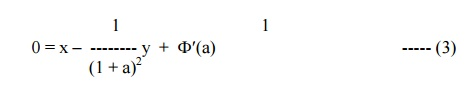Example 10

Solve p2 + q2 = npq

The solution of this equation is  z = ax + by + c, where a2 + b2 = nab.

Solving, we getDifferentiating (1) partially w.r.t c, we get  0 = 1, which is absurd. Therefore, there is no singular integral for the given equation.

To find the general Integral,    put C = F (a), we getThe eliminant of ŌĆ×aŌĆ¤ between these equations gives the general integral

Standard II : Equations of the form f (x,p,q) = 0, f (y,p,q) = 0 and f (z,p,q) = 0. i.e, one of the variables x,y,z occurs explicitly.

(i)                Let us consider the equation f (x,p,q) = 0.

Since z is a function of x and y, we have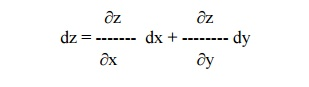or        dz = pdx + qdy

Assume that q = a.

Then the given equation takes the form f (x, p,a ) = 0

Solving, we get   p = F(x,a).

Therefore,        dz = F(x,a) dx + a dy.

(ii) Let us consider the equation f(y,p,q) = 0. Assume that p = a.

Then the equation becomes f (y,a, q) = 0 Solving, we get q = F (y,a).

Therefore, dz = adx + F(y,a) dy.

Integrating, z = ax + ├▓F(y,a) dy + b, which is a complete  Integral.

(iii) Let us consider the equation f(z, p, q) = 0.

Assume that q = ap.

Then the equation becomes f (z, p, ap) = 0

Solving, we get p = F(z,a). Hence dz = F(z,a) dx + a F(z, a) dy.Example 11

Solve q = xp + p2

Given q = xp + p2   -------------(1)

This is of the form f (x,p,q) = 0.

Put q = a in (1), we get

a = xp + p2

i.e, p2 + xp ŌĆōa = 0.

Therefore,Example 12

Solve q = yp2

This is of the form f (y,p,q) = 0

Then, put p = a.

Therfore, the given equation becomes q = a2y.

Since dz = pdx + qdy, we have

dz = adx + a2y dy

Integrating, we get z = ax + (a2y2/2) + b

Example 13

Solve 9 (p2z + q2) = 4

This is of the form f (z,p,q) = 0

Then, putting       q = ap, the given  equation becomes

9 (p2z + a2p2) = 4or      (z + a2)3/2 = x + ay + b.

Standard III : f1(x,p) = f2 (y,q). ie, equations in which ŌĆśzŌĆÖ is absent and the variables are separable.

Let us assume as a trivial solution that

f(x,p) = g(y,q) = a (say).

Solving for p and q, we get p = F(x,a) and q = G(y,a).Hence dz = pdx + qdy = F(x,a) dx + G(y,a) dy

Therefore, z = ├▓F(x,a) dx + ├▓G(y,a) dy + b , which is the complete integral of the given equation containing two constants a and b. The singular and general integrals are found in the usual way.

Example 14

Solve pq = xy

The given equation can be written asExample 15

Solve p2 + q2 = x2 + y2

The given equation can be written as p2 ŌĆōx2 = y2 ŌĆōq2 = a2 (say)

p2 ŌĆōx2 = aImplies p = ├¢(a2 + x2)

andy2 ŌĆōq2 = aImplies q = ├¢(y2 ŌĆōa2)

But   dz = pdx + qdyStandard IV (ClairautŌĆÖs) form

Equation of the type z = px + qy + f (p,q) ------(1) is   known   as   ClairautŌĆ¤s

Differentiating (1) partially w.r.t x and y, we get p = a and q = b.

Therefore, the complete integral is given by

z = ax + by + f (a,b).

Example 16

Solve z = px + qy +pq

The given equation is   in   ClairautŌĆ¤s.   form

Putting p = a and q = b, we have

z = ax + by + ab                                         -------- (1)

which is the complete integral.

To find the singular integral, differentiating (1) partially w.r.t a and b, we get

0 = x + b

0 = y + a

Therefore we have, a = -y and b= -x.

Substituting the values of a & b in (1), we get

z = -xy ŌĆōxy + xy

or      z + xy = 0,  which is the singular integral.

To get the general integral, put b = F(a) in (1).

Then z = ax + F(a)y + a F(a)                              ---------- (2)

Differentiating (2) partially w.r.t a, we have

0 = x + F'(a) y + aF'(a) + F(a)                  ---------- (3)

Eliminating ŌĆ×aŌĆ¤ between (2) and (3), we get the general integral.

Example 17

Find the complete and singular solutions of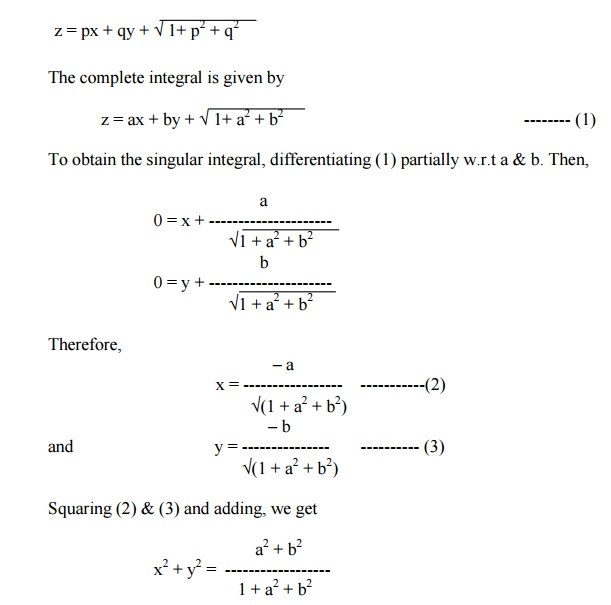Exercises

Solve the following Equations

1.     pq = k

2.     p + q = pq

3.     ├¢p +├¢q = x

4.     p = y2q2

5.     z = p2 + q2

6.     p + q = x + y

7.     p2z2  + q2 = 1

8.     z = px + qy - 2├¢pq

9.     {z ŌĆō(px + qy)}2 = c2 + p2 + q2

10.                        z = px + qy + p2q2

EQUATIONS REDUCIBLE TO THE STANDARD FORMS

Sometimes, it is possible to have non ŌĆōlinear partial differential equations of the first order which do not belong to any of the four standard forms discussed earlier. By changing the variables suitably, we will reduce them into any one of the four standard forms.

Type (i) : Equations of the form F(xm p, ynq) = 0 (or) F (z, xmp, ynq) = 0.

Case(i) : If m ┬╣1 and n ┬╣1, then put x1-m = X and y1-n = Y.Hence, the given equation takes the form F(P,Q) = 0 (or) F(z,P,Q) = 0.

Case(ii) : If m = 1 and n = 1, then put log x = X and log y = Y.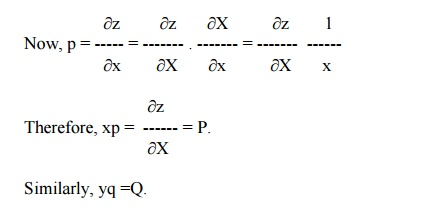Example 18

Solve x4p2 + y2zq = 2z2

The given equation can be expressed as

(x2p)2 + (y2q)z = 2z2

Here m = 2, n = 2

Put X = x1-m = x -1  and  Y = y 1-n = y -1.

We have xmp = (1-m) P  and ynq = (1-n)Q

i.e, x2p = -P and  y2q = -Q.

Hence the given equation becomes

P2 ŌĆōQz = 2z----------(1)

This equation is of the form f (z,P,Q) = 0.

Let us take Q = aP.

Then equation (1) reduces to

P2 ŌĆōaPz =2z2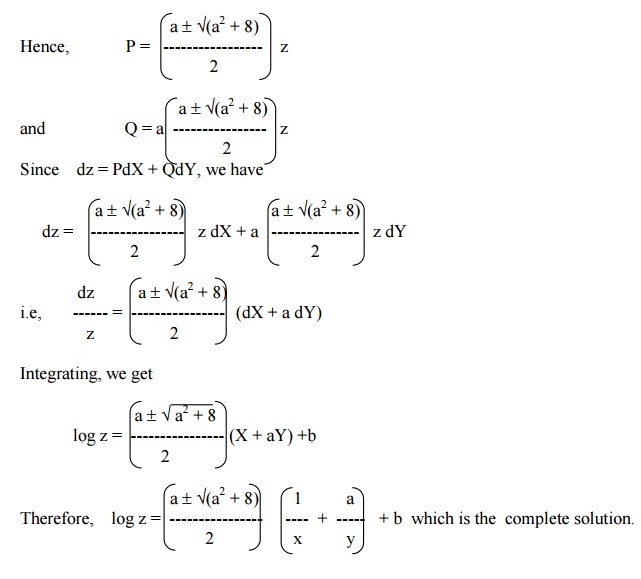Example 19

Solve x2p2 + y2q2  = z2

The given equation can be written as

(xp)2 + (yq)2  = z2

Here m = 1, n = 1.

Put X = log x  and Y = log y.

Then xp = P  and yq = Q.

Hence the given equation becomes

P2 + Q2  = z2   ------------- (1)

This equation is of the form F(z,P,Q) = 0.

Therefore, let us assume that Q = aP.

Now, equation (1) becomes,

P2 + a2 P2 = z2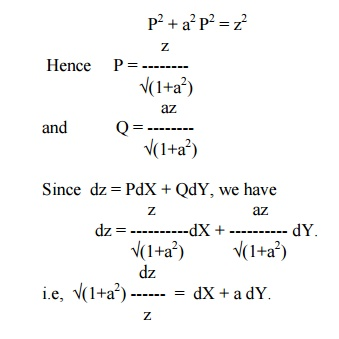Integrating, we get

├¢(1+a2) log z = X + aY + b.

Therefore, ├¢(1+a2) log z = logx + alogy + b, which is the complete solution.

Type (ii) : Equations of the form F(zkp, zkq) = 0 (or) F(x, zkp) = G(y,zkq).

Case (i) : If k ┬╣-1, put Z = zk+1,Example 20

Solve z4q2 ŌĆōz2p = 1

The given equation can also be written as

(z2q)2 ŌĆō(z2p) =1

Here k = 2. Putting Z = z k+1 = z3, we geti.e,   Q2 ŌĆō3P ŌĆō9 = 0,

which is of the form F(P,Q) = 0.

Hence its solution is Z = ax + by + c, where b2 ŌĆō3a ŌĆō9 = 0.

Solving for b,  b = ┬▒ ├¢(3a +9)

Hence the complete solution is

Z = ax  + ├¢(3a +9) . y + c

or      z3 = ax  + ├¢(3a +9) y + cStudy Material, Lecturing Notes, Assignment, Reference, Wiki description explanation, brief detail
Mathematics (maths) : Partial Differential Equations : Solution of a Partial Differential Equation |